Tamil Nadu Board of Secondary EducationHSC Science Class 11th

# Tamil Nadu Board Samacheer Kalvi solutions for Class 11th Chemistry Volume 1 and 2 Answers Guide chapter 14 - Haloalkanes and Haloarenes [Latest edition]

#### Chapters## Chapter 14: Haloalkanes and Haloarenes

Evaluation
Evaluation [Pages 251 - 256]

### Tamil Nadu Board Samacheer Kalvi solutions for Class 11th Chemistry Volume 1 and 2 Answers Guide Chapter 14 Haloalkanes and Haloarenes Evaluation [Pages 251 - 256]

Evaluation | Q I. 1. | Page 251

The IUPAC name of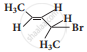is

• 2 – Bromo pent – 3 – ene

• 4 – Bromo pent – 2 – ene

• 2 – Bromo pent – 4 – ene

• 4 – Bromo pent – 1 – ene

Evaluation | Q I. 2. | Page 251

Of the following compounds, which has the highest boiling point?

• n – Butyl chloride

• Isobutyl chloride

• t – Butyl chloride

• n – Propyl chloride

Evaluation | Q I. 3. | Page 251

Arrange the following compounds in increasing order of their density

1. CCl4
2. CHCl3
3. CH2Cl2
4. CH3Cl
• D < C < B < A

• C > B > A > D

• A < B < C < D

• C > A > B > D

Evaluation | Q I. 4. | Page 251

With respect to the position of – Cl in the compound CH3 – CH = CH – CH2 – Cl, it is classified as ______.

• Vinyl

• Allyl

• Secondary

• Aralkyl

Evaluation | Q I. 5. | Page 251

What should be the correct IUPAC name of diethyl chloromethane?

• 3 – Chloro pentane

• 1 – Chloropentane

• 1 – Chloro – 1, 1 – diethyl methane

• 1- Chloro-1-ethyl propane

Evaluation | Q I. 6. | Page 252

C -X bond is strongest in ______.

• Chloromethane

• Iodomethane

• Bromomethane

• Fluoromethane

Evaluation | Q I. 7. | Page 252

In the reaction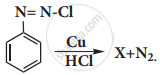. X is _______.

•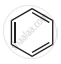•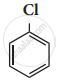•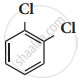•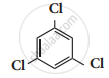Evaluation | Q I. 8. | Page 252

Which of the following compounds will give racemic mixture on nucleophilic substitution by OH- ion?

1. $\begin{array}{cc}\ce{CH3 - CH - CH2Br}\\ |\phantom{....}\\\ce{C2H5}\end{array}$
2. $\begin{array}{cc}\ce{CH3}\\ |\phantom{.}\\\ce{H3C - C - C2H5}\\ |\phantom{.}\\\ce{Br}\end{array}$
3. $\begin{array}{cc}\ce{H}\\ |\phantom{.}\\\ce{CH3 - C - C2H5}\\ |\phantom{.}\\\ce{Cl}\end{array}$
• (i)

• (ii) and (iii)

• (iii)

• (i) and (ii)

Evaluation | Q II. 9. | Page 252

The treatment of ethyl formate with excess of RMgX gives

• $\begin{array}{cc}\ce{R - C - R}\\||\\\ce{O}\end{array}$

• $\begin{array}{cc}\ce{R - CH - R}\\|\\\phantom{..}\ce{OH}\end{array}$

• R- CHO

• R- O – R

Evaluation | Q I. 10. | Page 252

Benzene reacts with Cl2 in the presence of FeCl3 and in absence of sunlight to form ______.

• Chlorobenzene

• Benzyl chloride

• Benzal chloride

• Benzene hexachloride

Evaluation | Q I. 11. | Page 252

The name of C2F4C12 is ______.

• Freon – 112

• Freon – 113

• Freon – 114

• Freon – 115

Evaluation | Q I. 12. | Page 252

Which of the following reagent is helpful to differentiate ethylene dichloride and ethylidene chloride?

• Zn/methanol

• KOH/ethanol

• aqueous KOH

• ZnCl2/Con HCl

Evaluation | Q I. 13. | Page 252

Match the compounds given in Column I with suitable items given in Column II.

 Column I (Compound) Column II (Uses) A Iodoform 1 Fire extinguisher B Carbon tetra chloride 2 Insecticide C CFC 3 Antiseptic D DDT 4 Refrigerants
• A → 2, B → 4, C →1, D →3

• A → 3, B → 2, C →4, D →1

• A → 1, B → 2, C → 3, D → 4,

• A → 3 B → 1 C → 4 D → 2

Evaluation | Q I. 14. | Page 253

Assertion: In mono haloarenes, electrophilic substitution occurs at ortho and para positions.

Reason: Halogen atom is a ring deactivator.

• If both assertion and reason are true and reason is the correct explanation of assertion.

• If both assertion and reason are true but reason is not the correct explanation of assertion.

• If assertion is true but reason is false.

• If both assertion and reason are false.

Evaluation | Q I. 15. | Page 253

Consider the reaction,

$\ce{CH3CH2CH2Br + NaCN → CH3CH2CH2CN + NaBr}$

This reaction will be the fastest in

• ethanol

• b) methanol

• DMF (N, N’ – dimethyl formamide)

• water

Evaluation | Q I. 16. | Page 253

Freon-12 is manufactured from tetrachloro methane by ______.

• Wurtz reaction

• Swarts reaction

• Haloform reaction

• Gattermann reaction

Evaluation | Q I. 17. | Page 253

The most easily hydrolysed molecule under SN1 condition is ______.

• allyl chloride

• ethyl chloride

• ispropylchloride

• benzyl chloride

Evaluation | Q I. 18. | Page 253

The carbocation formed in SN1 reaction of alkyl halide in the slow step is ______.

• sp3 hybridized

• sp2 hybridized

• sp hybridized

• none of these

Evaluation | Q I. 19. | Page 253

The major products obtained when chlorobenzene is nitrated with HNO3 and con H2SO4

• 1 – chloro – 4 – nitrobenzene

• 1 – chloro – 2 – nitrobenzene

• 1 – chloro – 3 – nitrobenzene

• 1 – chloro – 1 – nitrobenzene

Evaluation | Q I. 20. | Page 253

Which one of the following is most reactive towards nucleophilic substitution reaction?

•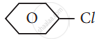•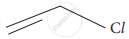•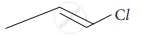•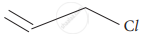Evaluation | Q I. 21. | Page 253

Ethylidene chloride on treatment with aqueous KOH gives ______.

• acetaldehyde

• ehtyleneglycol

• formaldehyde

• glycoxal

Evaluation | Q I. 22. | Page 253

The raw material for Raschig process

• chloro benzene

• phenol

• benzene

• anisole

Evaluation | Q I. 23. | Page 254

Chloroform reacts with nitric acid to produce ______.

• nitro toluene

• nitro glycerine

• chloropicrin

• chloropicric acid

Evaluation | Q I. 24. | Page 254

$\ce{acetone ->[i) CH3MgI][ii) H2O/H^{-1}]}$X, X is ______.

• 2 – propanol

• 2 – methyl – 2 – propanol

• 1 – propanol

• acetonol

Evaluation | Q I. 25. | Page 254

Silver propionate when refluxed with Bromine in carbon tetrachloride gives ______.

• propionic acid

• chloroethane

• Bromo ethane

• chloro propane

#### Write brief answer to the following questions.

Evaluation | Q II. 1. i) | Page 254

Classify the following compound in the form of alkyl, allylic, vinyl, benzylic halides.

$\ce{CH3 – CH = CH – Cl}$

Evaluation | Q II. 1. ii) | Page 254

Classify the following compound in the form of alkyl, allylic, vinyl, benzylic halides.

C6H5CH2I

Evaluation | Q II. 1. iii) | Page 254

Classify the following compound in the form of alkyl, allylic, vinyl, benzylic halides.

$\begin{array}{cc}\ce{CH3 - CH - CH3}\\ |\phantom{..}\\\ce{Br}\end{array}$

Evaluation | Q II. 1. iv) | Page 254

Classify the following compound in the form of alkyl, allylic, vinyl, benzylic halides.

CH2 = CH – Cl

Evaluation | Q II. 2. | Page 254

Why chlorination of methane is not possible in dark?

Evaluation | Q II. 3. | Page 254

How will you prepare n propyl iodide from n-propyl bromide?

Evaluation | Q II. 4. | Page 254

Which alkyl halide from the following pair is

1. chiral
2. undergoes faster SN2 reaction?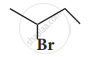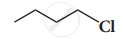Evaluation | Q II. 5. | Page 254

How does chlorobenzene react with sodium in the presence of ether? What is the name of the reaction?

Evaluation | Q II. 6. | Page 254

Give reasons for the polarity of C – X bond in haloalkane.

Evaluation | Q II. 7. | Page 254

Why is it necessary to avoid even traces of moisture during the use of Grignard reagent?

Evaluation | Q II. 8. | Page 254

What happens when acetyl chloride is treated with an excess of CH3MgI?

Evaluation | Q II. 9. | Page 254

Arrange the following alkyl halide in increasing order of bond enthalpy of RX.

CH3Br, CH3F, CH3Cl, CH3I

Evaluation | Q II. 10. | Page 254

What happens when chloroform reacts with oxygen in the presence of sunlight?

Evaluation | Q II. 11. | Page 254

Write down the possible isomers of C5H11Br and give their IUPAC and common names.

Evaluation | Q II. 12. | Page 254

Mention any three methods of preparation of haloalkanes from alcohols.

Evaluation | Q II. 13. | Page 254

Compare SN1 and SN2 reaction mechanisms.

Evaluation | Q II. 14. | Page 254

Reagents and the conditions used in the reactions are given below. Complete the table by writing down the product and the name of the reaction.

 Reaction Product Name of the reaction $\ce{CH3CH2OH + SOCl2 ->[pyridine] ?}$ ______ ______ $\ce{CH3CH2Br + AgF ->}$ ? ______ ______ $\ce{C6H5Cl + Na ->[Ether]}$ ______ ______
Evaluation | Q II. 15. | Page 254

Discuss the aromatic nucleophilic substitutions reaction of chlorobenzene.

#### Account for the following

Evaluation | Q II. 16. (i) | Page 255

t – butyl chloride reacts with aqueous KOH by SN1 mechanism while n – butyl chloride reacts with SN2 mechanism.

Evaluation | Q II. 16. (ii) | Page 255

p – dichlorobenzene has a higher melting point than those of o – and m – dichlorobenzene.

Evaluation | Q II. 17. | Page 255

In an experiment ethyliodide in ether is allowed to stand over magnesium pieces. Magnesium dissolves and product is formed

1. Name the product and write the equation for the reaction.
2. Why all the reagents used in the reaction should be dry? Explain.
3. How is acetone prepared from the product obtained in the experiment.
Evaluation | Q II. 18. i) | Page 255

Write a chemical reaction useful to prepare the following:

Freon – 12 from Carbon tetrachloride

Evaluation | Q II. 18. ii) | Page 255

Write a chemical reaction useful to prepare the following:

Carbon tetrachloride from carbon disulphide.

Evaluation | Q II. 19. a. | Page 255

What are Freons?

Evaluation | Q II. 19. b. | Page 255

Discuss uses of ferons.

Evaluation | Q II. 19. c, | Page 255

Discuss the environmental effects of freons.

Evaluation | Q II. 20. i) | Page 255

Predict the product when Bromo ethane is treated with the following.

KNO2

Evaluation | Q II. 20. ii) | Page 255

Predict the products when Bromo ethane is treated with the following.

AgNO2

Evaluation | Q 21. | Page 254

Explain the mechanism of SN1 reaction by highlighting the stereochemistry behind it.

Evaluation | Q II. 22. i) | Page 255

Write a short note on raschig process.

Evaluation | Q II. 22. ii) | Page 255

Write a short note on Dows Process.

Evaluation | Q II. 22. iii) | Page 255

Write a short note on Darzen’s process.

Evaluation | Q II. 23. i) | Page 255

Starting from CH3MgI, How will you prepare the following?

Acetic acid

Evaluation | Q II. 23. ii) | Page 255

Starting from CH3MgI, How will you prepare the following?

Acetone

Evaluation | Q II. 23. iii) | Page 255

Starting from CH3MgI, How will you prepare the following?

Ethyl acetate

Evaluation | Q II. 23. iv) | Page 255

Starting from CH3MgI, How will you prepare the following?

Iso propyl alcohol

Evaluation | Q II. 23. v) | Page 255

Starting from CH3MgI, How will you prepare the following?

Methyl cyanide

Evaluation | Q II. 24. i) | Page 255

Complete the following reaction.

$\ce{CH3 - CH = CH2 + HBr ->[Peroxide]}$

Evaluation | Q II. 24. ii) | Page 255

Complete the following reaction.

$\ce{CH3 - CH2 - Br + NaSH ->[alcohol][H2O]}$

Evaluation | Q II. 24. iii) | Page 255

Complete the following reaction.

$\ce{C6H5Cl + Mg ->[THF]}$

Evaluation | Q II. 24. iv) | Page 255

Complete the following reaction.

$\ce{CHCl3 + HNO3 ->[\Delta]}$

Evaluation | Q II. 24. v) | Page 255

Complete the following reaction.

$\ce{CCl4 + H2O ->[\Delta]}$

Evaluation | Q II. 25. i) | Page 255

Explain the preparation of the following compound.

DDT

Evaluation | Q II. 25. ii) | Page 255

Explain the preparation of the following compound.

Chloroform

Evaluation | Q II. 25. iii) | Page 256

Explain the preparation of the following compound.

Biphenyl

Evaluation | Q II. 25. (iv) | Page 256

Explain the preparation of the following compound.

Chloropicrin

Evaluation | Q II. 25. (v) | Page 256

Explain the preparation of the following compound.

Freon-12

Evaluation | Q II. 26. | Page 256

An organic compound (A) with molecular formula C2H5Cl reacts with KOH gives compounds (B) and with alcoholic KOH gives compound (C). Identify (A), (B), (C).

Evaluation | Q II. 27. | Page 256

The simplest alkene (A) reacts with HCl to form a compound (B). Compound (B) reacts with ammonia to form compound (C) of molecular formula C2H7N. Compound (C) undergoes carbylamine test. Identify (A),
(B) and (C).

Evaluation | Q II. 28. | Page 256

A hydrocarbon C3H6(A) reacts with HBr to form compound (B). Compound (B) reacts with aqueous potassium hydroxide to give (C) of molecular formula C3H6O. What are the (A), (B) and (C). Explain the reactions.

Evaluation | Q II. 29. | Page 256

Two isomers (A) and (B) have the same molecular formula C2H4Cl2. Compound (A) reacts with aqueous KOH gives compound (C) of molecular formula C2H4O. Compound (B) reacts with aqueous KOH gives compound (D) of molecular formula C2H6O2. Identify (A), (B), (C) and (D).

## Chapter 14: Haloalkanes and Haloarenes

Evaluation## Tamil Nadu Board Samacheer Kalvi solutions for Class 11th Chemistry Volume 1 and 2 Answers Guide chapter 14 - Haloalkanes and Haloarenes

Tamil Nadu Board Samacheer Kalvi solutions for Class 11th Chemistry Volume 1 and 2 Answers Guide chapter 14 (Haloalkanes and Haloarenes) include all questions with solution and detail explanation. This will clear students doubts about any question and improve application skills while preparing for board exams. The detailed, step-by-step solutions will help you understand the concepts better and clear your confusions, if any. Shaalaa.com has the Tamil Nadu Board of Secondary Education Class 11th Chemistry Volume 1 and 2 Answers Guide solutions in a manner that help students grasp basic concepts better and faster.

Further, we at Shaalaa.com provide such solutions so that students can prepare for written exams. Tamil Nadu Board Samacheer Kalvi textbook solutions can be a core help for self-study and acts as a perfect self-help guidance for students.

Concepts covered in Class 11th Chemistry Volume 1 and 2 Answers Guide chapter 14 Haloalkanes and Haloarenes are Introduction to Haloalkanes and Haloarenes, Classification of Organic Halogen Compounds, Haloalkanes, Organo Metallic Compounds, Haloarenes, Poly Halogen Compounds.

Using Tamil Nadu Board Samacheer Kalvi Class 11th solutions Haloalkanes and Haloarenes exercise by students are an easy way to prepare for the exams, as they involve solutions arranged chapter-wise also page wise. The questions involved in Tamil Nadu Board Samacheer Kalvi Solutions are important questions that can be asked in the final exam. Maximum students of Tamil Nadu Board of Secondary Education Class 11th prefer Tamil Nadu Board Samacheer Kalvi Textbook Solutions to score more in exam.

Get the free view of chapter 14 Haloalkanes and Haloarenes Class 11th extra questions for Class 11th Chemistry Volume 1 and 2 Answers Guide and can use Shaalaa.com to keep it handy for your exam preparation